Impact Factor 4.677 | CiteScore 5.4
More on impact ›

# Frontiers in Neuroscience

## ORIGINAL RESEARCH article

Front. Neurosci., 06 March 2012 | https://doi.org/10.3389/fnins.2012.00029

# Decoding finger movements from ECoG signals using switching linear models

• LITIS EA 4108 – INSA, Université de Rouen, Saint Etienne du Rouvray, France

One of the most interesting challenges in ECoG-based Brain-Machine Interface is movement prediction. Being able to perform such a prediction paves the way to high-degree precision command for a machine such as a robotic arm or robotic hands. As a witness of the BCI community increasing interest toward such a problem, the fourth BCI Competition provides a dataset which aim is to predict individual finger movements from ECoG signals. The difficulty of the problem relies on the fact that there is no simple relation between ECoG signals and finger movements. We propose in this paper, to estimate and decode these finger flexions using switching models controlled by an hidden state. Switching models can integrate prior knowledge about the decoding problem and helps in predicting fine and precise movements. Our model is thus based on a first block which estimates which finger is moving and another block which, knowing which finger is moving, predicts the movements of all other fingers. Numerical results that have been submitted to the Competition show that the model yields high decoding performances when the hidden state is well estimated. This approach achieved the second place in the BCI competition with a correlation measure between real and predicted movements of 0.42.

## 1. Introduction

Some people who suffer neurological diseases can become severely impaired and have strongly reduced motor functions but still have some cognitive abilities. One of their possible way to communicate with their environment is by using their brain activities. Brain-Computer interfaces (BCI) research aim at developing systems to help such disabled people communicating with other people through machines. Non-invasive BCIs have recently received a lot of interest because of their easy protocol for sensor implantation on the scalp surface (Blankertz et al., 2004; Wolpaw and McFarland, 2004). Furthermore, although the electroencephalogram signals have been recorded through the skull and are known to have poor Signal-to-Noise Ratio (SNR), those BCI have shown great capabilities, and have already been considered for daily use by Amyotrophic Lateral Sclerosis (ALS) patients (Sellers and Donchin, 2006; Nijboer et al., 2008; Sellers et al., 2010).

However, non-invasive recordings still show some drawbacks including poor signal-to-noise ratio and poor spatial resolution. Hence, in order to overcome these issues, invasive BCI may instead be considered. For instance, Electrocorticographic recordings (ECoG) have recently received a great amount of attention owing to their semi-invasive nature as they are recorded from the cortical surface. They offer high spatial resolution and they are far less sensitive to artifact noise. Feasibility of invasive-based BCI have been proven by several recent works (Leuthardt et al., 2004; Hill et al., 2006, 2007; Shenoy et al., 2008). Yet, most of these papers consider motor imagery as a BCI paradigm and thus do not take advantage of the fine degree of control that can be gained with the ECoG signals.

Achieving such high degree of control is an important challenge for BCI since it would make possible the control of a cursor, a mouse pointer, or a robotic arm (Wolpaw et al., 1991; Krusienski et al., 2007). Toward this aim, a recent breakthrough has been made by Schalk et al. (2007) proving that ECoG recordings can lead to continuous BCI control with multiple degree of freedom. Along with the work of Pistohl et al. (2008), they have studied the problem of predicting real arm movements from ECoG signals. As such, it is important to note that unlike other BCIs, real movements are considered in these works, hence the global approaches they propose do not suit to impaired subjects.

Following the road paved by Schalk et al. (2007) and Pistohl et al. (2008), we investigate in this work, the feasibility of a fine degree of resolution in BCI control by addressing the problem of estimating finger flexions through ECoG signals. Indeed, we propose in this paper a method for decoding finger movements from ECoG data based on switching models. The underlying idea of these switching models is based on the hypothesis that movements of each of the five fingers are triggered by an internal discrete state that can be estimated and that all finger movements depend on that internal state. While such an idea of switching models have already been successfully used for arm movement prediction on monkeys, based on micro-electrode array measures (Darmanjian et al., 2006), here we develop a specific approach adapted to finger movements. The global method has been tested and evaluated on the 4th Dataset of the BCI Competition IV (Miller and Schalk, unpublished) yielding to a second place in the competition.

The paper is organized as follows: first, we briefly present the BCI Competition IV dataset used in this paper for evaluating our method and provide an overview of the global decoding method. Then we delve into the details of the proposed switching models for finger movement prediction from ECoG signals. Finally, we present numerical experiments designed for evaluating our contribution followed by a discussion about the limits of our approach and about future works.

## 2. Dataset

In this work, we focus on the fourth dataset from the BCI Competition IV (Miller and Schalk, unpublished). The task related to this dataset is to predict finger flexions and finger extensions from signals recorded on the surface of the brain of the subjects. In what follows, we use the term “flexion” for both kinds of movements as in the dataset description (Miller and Schalk, unpublished). The signals composing the dataset, have been acquired from three epileptic patients who had platinium electrode grids placed on the surface of their brain, the number of electrodes varying between 48 and 64 depending on the subject. Note that the electrode positions on the cortex have not been provided by the competition organizer impeding thus the use of spatial prior knowledge such as electrode’s neighborhood for building the finger movement model.

Electrocorticographic (ECoG) signals of the subjects were recorded at a 1-kHz sampling using BCI2000 (Schalk et al., 2004). A band-pass filter from 0.15 to 200 Hz was applied to the ECoG signals. The finger flexion of the subject was recorded at 25 Hz and up-sampled to 1 kHz. Note that the finger flexion signals have been normalized with zero mean an unit variance. Due to the acquisition process, a delay appears between the finger movement and the measured ECoG signal. To correct this time-lag, we apply a 37-ms delay to the ECoG signals (Miller and Schalk, unpublished) as suggested in the dataset description.

The full BCI Competition dataset consists in a 10-min recording per subject. Six minutes 40 s (400,000 samples) were given to the contestants for learning the finger movement models and the remaining 3 min 20 s (200,000 samples) were used for evaluating the model. Since the finger flexion signals have been up-sampled and thus are partly composed of artificial samples, we have down-sampled the number of points by a factor of 4 leading to a training set of size 100,000 and a testing set of size 50,000. The 100,000 samples provided for learning have been split in a training (75,000) and validation set (25,000). Note that all parameters in the proposed approach have been selected in order to minimize the error on the validation set. All results presented in the paper have been obtained on the testing set provided by the competition.

In this competition, performances of methods proposed by competitors were evaluated according to the correlation between measured and predicted finger flexions. These correlations were averaged across fingers and across subjects to obtain the overall method performance. However, because its movements were highly physically correlated with those of the other finger, the fourth finger is not included in the evaluation (Miller and Schalk, unpublished).

## 3. Finger Flexion Decoding Using Switching Linear Models

This section presents our decoding method for addressing the problem of estimating finger movement from ECoG signals. At first, we introduce a global view of the model and briefly discuss the decoding scheme. The second part of the section is devoted to a detailed description of each block of the switching model: the internal state estimation, the linear finger prediction, and the final decoding stage.

### 3.1. Overview

The main idea on which our switching model used for predicting finger movement from ECoG signals is built, is the assumption that measured ECoG signals and finger movements are intrinsically related to one or several internal states.

These internal states play a central role in our model. Indeed, it allows us to learn from training examples a specific model associated to each single state. In this sense, the complexity of our model depends on the number of possible hidden states: if only one hidden state is possible then all finger movements would be predicted by a single model. If more hidden states are allowed, then we can build more specific models related to specific states of the ECoG signals. According to this, allowing too many hidden states may thus lead to a global model that overfits the training data.

In the learning problem addressed in here, the ECoG signals present some specificities. Indeed, during the acquisition process, subjects have been dictated to move only one finger at a time, thus it appeared natural to us to take profit of this prior knowledge for building a better model. Hence, we have considered an internal state k that can take six different values, depending on which finger is moving: k = 1 for the thumb to k = 5 for the baby finger or k = 6 for no finger movement. This is in accordance with the experimental set-up where mutually exclusive states are in play, nonetheless for another dataset where any number of finger can move simultaneously, we can use another model for the hidden states, e.g., one binary state per finger corresponding to movement or no movement.

Figure 1 summarizes the big picture of our finger movement decoding scheme. Basically, the idea is that based on some features extracted from the ECoG signals, the internal hidden state triggering the switching finger models can be estimated. Then, this state k controls a linear switching model of parameters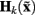that will predict the finger movements.

FIGURE 1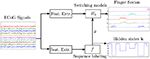Figure 1. Diagram of the switching models decoder. We see that two models ares estimated from the ECoG signals: (bottom flow) one which outputs a state k predicting which finger is moving and (top flow) another one that, given the predicted moving finger, estimates the flexion of all fingers.

According to this global scheme, we need to estimate the function f(·) that maps the ECoG features to an internal state k ∈ {1, …, 6} and estimate the parameter of the linear model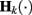that relates the brain signals to the movements of all fingers. The next paragraphs introduce these functions and clarify how they have been learned from training data.

### 3.2. Moving Finger Estimation

The proposed switching model requires the estimation of an hidden state. In our application, the hidden state k is a discrete state representing which finger is moving. Learning a model f(·) that estimates these internal states can be interpreted as a sequence labeling problem. There exists several sequence labeling approaches in the literature such as HMM or CRF. Nevertheless, those methods require an offline decoding, typically based on the well-known Viterbi algorithm, which precludes their applications for online movement prediction. We propose in the sequel to use a simple time sample classification scheme for solving this sequence labeling problem.

In the following, we depict the features and the methodology used for learning the function f(·) that predicts the value of this internal state.

#### Feature extraction

We used smoothed Auto-Regressive (AR) coefficients of the signal as features because they capture some dynamics of the signals. The global overview of the feature extraction procedure is given in Figure 2. For a single channel, the procedure is the following. The signal is divided in non-overlapping windows of 300 samples. For each window, an auto-regressive model is estimated. Thus, AR coefficients are obtained at every 300 samples (denoted by the vertical dashed line and the cross in Figure 2). In order to have continuous values of the AR coefficients, a smoothing spline-based interpolation between two consecutive AR coefficients is applied. Note that instead of interpolating, we could have computed the AR coefficients at each time instant, however, this heuristic has the double advantage of being computationally less demanding and of providing some smoothed (and thus more robust to noise) AR coefficients. For computational reasons, only the two first AR coefficients of a model of order 10 are used as features. Indeed, we find out from a validation procedure that among all the AR coefficients, the two first ones are the most discriminative. Further signal dynamics is taken into account by applying a similar procedure to shifted versions of the signal at (+ts and −ts), which multiplies the number of features by 3. Note that by using a positive lag +ts, our feature extraction approach becomes non-causal which in principle, would preclude its use for real-time BCI. Nevertheless, this lag does not exceed the window size used for AR feature extraction and thus it has limited impact on the delay of the decision process, while it considerably enhances the system performances.

FIGURE 2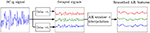Figure 2. Diagram of the feature extraction procedure for the moving finger decoding. Here, we have outlined the processing of a single channel signal.

To summarize, for measurements involving 48 channels, the feature vector at a time instant t is obtained by concatenating the six AR features extracted from each single channel, leading to a resulting vector of size 48 × 3 × 2 = 240.

#### Channel selection

Actually, some channels are not used in the function f(·), since we decided to perform a simple channel selection to keep only the most relevant ones. For us, the channel selection has two objectives: (i) to substantially reduce the number of channels and thus to minimize the computational effort needed for estimating and evaluating the function f(·) and (ii) to keep in the model only the most relevant channels so as to increase estimation performances. For this channel selection procedure, the feature vector x t at time t has been computed as described above, except that for computational reasons, we do not have considered the shifted signal versions and use only the first AR coefficient. Again, we experienced on the validation set that these features were sufficient for having a good estimate of the relevant channels.

Then, for each finger, we estimate a linear regression of the form x tc k, based on the training set {x t, yt}, where x t ∈ ℝchan is a feature vector of number of channels dimension and yt takes values +1 or −1 whether the considered finger is moving or not. Once the coefficient vectors c k for all finger are estimated, we compute the relevance score of each channel as: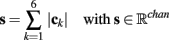where the absolute value is applied elementwise. The M relevant channels are those having the M largest score in the vector s, M being chosen in order to maximize the correlation on the validation set. This approach, although unusual, is highly related to a channel selection method based on a mixed-norm. Indeed, the above criterion can be understood as a ℓ0 − ℓ1 criterion where the ℓ0 selection would have been performed by comparing channels ℓ1 scores to an adaptive threshold dependent on M. Note that this channel selection scheme has also been successfully used for sensor selection in other competitions (Labbé et al., 2010).

#### Model estimation

The procedure for learning the function f(·) is as follows. First, since in this particular problem, the finger movements are mutually exclusive, we consider a winner-takes-all strategy and defines f(·) as: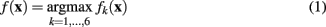where fk(·) are linear real-valued functions of the form fk(x) = xTck, that are trained so as to predict the presence or absence of finger movement for finger k. The features we use take into account some dynamics of the ECoG signals through the shifted features (+ts and −ts) and a finer feature selection has been performed by means of a simultaneous sparse approximation method as described in the sequel.

Let us consider the training examples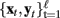where x t ∈ ℝd, with d = 240 and yt,k= {1, −1}, being the k-th entry of vector y t ∈ {1, −1}6, t denoting the time instant and k denoting the internal states of each finger. yt,k tells us whether the finger k = 1,…, 5 is moving at time t while yt,6 = 1 translates the fact that no fingers are moving at time t. Now, let us define the matrices Y, X, and C as: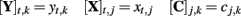where xt,j and cj,k are the j-th components of respectively x t and c k. The aim of simultaneous sparse approximation is to learn the coefficient matrix C while yielding the same sparsity profile in the different finger models. The task boils down to the following optimization problem: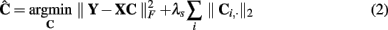where λs is a trade-off parameter that has to be properly tuned and C i, is the i-th row of C. Note that our penalty term is a mixed ℓ1 − ℓ2 norm similar to the one used for group-lasso (Yuan and Lin, 2006). Owing to the ℓ1 penalty on the ℓ2 row-norm, such a penalty tends to induce row-sparse matrix C. Problem (2) has been solved using the block-coordinate descent algorithm proposed by Rakotomamonjy (2011).

### 3.3 Learning Finger Flexion Models

We now discuss the model relating the ECoG signal and the finger movement amplitudes. This model is controlled by an internal state k, which means that we build an estimation of the movement of all fingers and the choice of the appropriate model then depends on the estimated internal state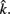Hence, for each value of the internal state k, we learn a linear model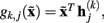with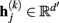being a vector of coefficients, that predicts the movement of finger j = 1,…, 5 when the finger k is moving. Note that obviously the movements of the fingers jk are quite small but different from zero as the finger movements are physically correlated. Linear model has been chosen since they proved to achieve good performances for decoding movements from ECoG signals (Schalk et al., 2007; Pistohl et al., 2008).

Feature extraction is performed following the same line as (Pistohl et al., 2008), i.e., we use filtered time samples as features. First, all channels are filtered with a Savitsky-Golay (third order, 0.4 s width) low-pass filter. Then, the feature vector at time t,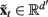is obtained by concatenating the time samples at t, t − τ, and t + τ for all smoothed signals and for all channels. Samples at t − τ and t + τ are used in order to take into account slight temporal delays between the brain activity and finger movements. Once more, our decoding method is thus not causal, but as only small values for τ are considered (≪1 s), the decision delay is reduced.

Let us now detail how the matrix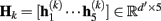containing the finger linear model parameters for state k, is learned. For each finger k, we extract all samples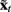when that finger is known to be moving. For this purpose, we have manually segmented the signals and extracted the appropriate signal segments, in order to build the target matrix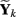(finger movement amplitude when finger k is moving), and the corresponding feature matrix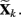. This training sample extraction stage is illustrated on Figure 3.

FIGURE 3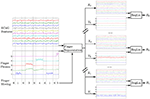Figure 3. Workflow of the learning sets extraction (Xk and Yk) and estimation of the coefficient matrix Hk.

Finally, the linear models for state k ∈ 1, …, 5 are learned by solving the following multi-dimensional ridge regression problem: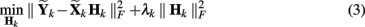with λk a regularization parameter that has to be tuned.

For this finger movement estimation problem, we also observed that feature selection helps in improving performances. Again, we use the estimated matrix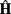coefficients for pruning the model,being the minimizer of equation (3). Similarly to the feature selection procedure introduced in section 2, we keep the M′ features having the largest entries of vector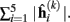M′ being chosen as to minimize the validation error.

### 3.4 Decoding Finger Movement

Once the linear models and the hidden state estimator are learned, we can apply the decoding scheme given in Figure 1. The decoding is a 2-step approach requiring the two feature vectors xt andused respectively for moving finger estimation and for estimating all finger flexion amplitudes. Estimated finger positions at time t is obtained by: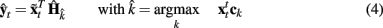with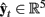a vector containing the estimated finger movements at time t,the estimated moving finger, and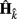the estimated linear model for state k.

## 4. Results

In this section, we discuss the performances of our switching model decoder. But at first, we explain how the different parameters the overall model have been selected. Next, we establish the soundness of linear models for finger movement prediction by evaluating their performance just on part of the test examples related to movements. Finally, we evaluate our approach on the complete data and compare its performances to those of other competitors.

### 4.1. Parameter Selection

All parameters used in the global model are selected by a validation method on the last part of the training set (75,000 for the training, 25,000 for validation). We suppose that the validation set size is large enough to avoid over-fitting. Examples of training and validation set sizes for each hidden state k are given in Table 1 for subject 1.

TABLE 1

Hence, for the learning function f(·), we select the number of relevant channels M, the time-lag ts used in feature extraction and the regularization term λs of equation (2) while for estimating H k, we tune the number of selected channels M′, τ, and λk. All these parameters are chosen so that they optimize the model performances on the validation set.

### 4.2. Linear Models Hk for Predicting Movement

Linear models are known to be accurate models for arm movement prediction (Schalk et al., 2007; Pistohl et al., 2008), here we want to confirm the hypothesis that their use can also be extended to finger movement predictions. For each finger k, we extract the ECoG signals and finger flexion amplitude when that finger is actually moving (see Figure 4) and we predict the finger movement on the test examples using the appropriate column of H k. The correlation between the predicted finger movement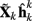and the actual movement y k is computed for each finger k of each subject. The results are shown in Table 2 and since they have been obtained only on samples where the actual finger is moving, they can not be compared to the competition results as they do not take into account part of the signals related to other finger movements.

FIGURE 4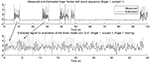Figure 4. Signal extraction for linear model estimation: (upper plot) full signal with segmented signal, corresponding to moving finger, bracketed by the vertical lines and (lower plot) the extracted signal corresponding to the concatenation of the samples when finger 1 is moving.

TABLE 2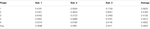Table 2. Correlation coefficient obtained by the linear models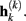.

We observe that by using a linear regression between the feature extracted from the ECoG signals and the finger flexions, we achieve a correlation of 0.46 (averaged across fingers and subjects). This results correspond to those obtained for the arm trajectory prediction (Schalk et al., 2007 obtained 0.5 and Pistohl et al., 2008 obtained 0.43). We can then conclude that the linear models provide an interesting baseline for finger movement predictions.

### 4.3. Switching Models for Movement Prediction

In order to evaluate the accuracy and the contributions of our the switching model, we report three different results: (a) we compute the estimated finger flexion using a unique linear model trained on all samples (including those ones where the considered finger is not moving), (b) we decode finger flexions with our switching decoder while assuming that the exact sequence of hidden states is known1, and (c) we use the proposed switching decoder with the estimated hidden states.

Correlation coefficients between real flexions and those predicted by the baseline model (a) are reported in Table 3 and predicted flexions can also be seen on the upper plots of Figure 5. We note that the correlations obtained are rather low (an average of 0.30). We conjecture that this is due to the fact that the model parameters are learned on the complete signal (which includes no movement). Indeed, the long temporal segments with small magnitudes have a tendency to shrink the global output toward zero. This is an issue that can be addressed by using our switching linear models.

FIGURE 5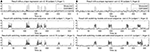Figure 5. True and estimated finger flexion for (upper plots) a global linear regression, (middle plots) switching decoder with true moving finger segmentation and (lower plots) with the switching decoder with an estimated moving finger segmentation. (A) Correspond to predictions of the first finger of subject 1 and (B) Correspond to the prediction of the second finger of subject 1.

TABLE 3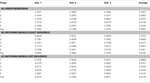Table 3. Correlation between measured and estimated movement for a global linear regression (A), switching decoder with exact sequence (B), and switching decoder with an estimated sequence (C).

The switching model decoder is a two-part process as it requires the linear models H k and the sequence of hidden states (see Figure 1). In order to evaluate the optimal performances of the switching model, we apply the decoder using the exact sequence k obtained from the actual finger flexion. We know that this cannot be done in practice as it would imply a perfect sequence labeling, but in our opinion, it gives an interesting idea of the potential of the switching models approach for given linear models H k. Examples of estimation can be seen in the middle plots of Figure 5 and while correlation coefficients are in Table 3. We obtain a high accuracy across all subjects with an average correlation of 0.61 when using an exact sequence. This proves that the switching model can be efficiently used for decoding ECoG signals.

Finally, we evaluate the switching models approach when using the finger moving estimator. In other words, we use the switching models H k to decode the signals with equation (4) and the estimated sequence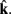The finger movement estimation can be seen on the lower plot of Figure 5B and the correlation measures are in Table 3. As expected, the accuracy is lower than those obtained with the true segmentation. However, we obtained an average correlation of 0.42 which is far better than the correlation obtained from a unique linear model. These predictions of the finger flexions were presented in the BCI Competition and achieved the second place. Note that the last 3 fingers have the lowest performances. Indeed, those fingers are highly physically correlated and much more difficult to discriminate than the two first ones in the sequence labeling. The first finger is by far the best estimated one as we obtained a correlation averaged across subject of 0.56.

### Discussion and Future Works

The results presented in the previous section have been submitted to the BCI Competition IV. We achieved the second place with an average correlation of 0.42 while the best performance have been obtained obtained by Liang and Bougrain, 2009; a correlation of about 0.46). Their method considers an amplitude modulation along time to cope with the abrupt change in the finger flexions magnitude along time. Such an approach is somewhat similar to ours since they try to distinguish between situations where fingers were moving or fingers were still.

We believe that our approach can be improved in several ways. Indeed, we chose to use linear models that are triggered by an internal state, while (Pistohl et al., 2008) proposed to use a Kalman filter for the movement decoding. Hence, it would be interesting to investigate whether Kalman filter or a non-linear model can help us in getting a better model.

Furthermore, our sequence labeling approach for estimating the sequence of hidden states can be also improved. Liang and Bougrain (2009) proposed to use Power Spectral Densities of the ECoG channel as features and we believe that the sequence labeling might benefit from the use of this kind of features. Finally, we have used a simple sequence labeling approach by doing a temporal sample classification of low-pass filtered features. Since other sequence labeling methods like Hidden Markov Models (Darmanjian et al., 2006) or Conditional Random Fields (Luo and Min, 2007) have been successfully proposed for BCI application, we believe that a more robust sequence labeling approach can increase the quality of the estimated segmentation and thus the final performances. For instance, the sequence SVM proposed by Bordes et al. (2008) can efficiently decode a sequence in real-time and has shown good predictive performances.

Finally, the question of how to predict statically held finger position is still open. Our approach has shown good finger movement estimations but when still, fingers were always at the same resting position, which is a favorable case. To address this problem of held finger, we can simply extend our global model by making the internal state estimator predict a static finger position.

## Conclusion

In this paper, we present a method for finger flexions prediction from ECoG signals. The decoder, based on switching linear models, has been evaluated in the BCI Competition IV Dataset 4 and achieved the second place in the competition. We show empirically the advantages of the switching models scheme over a unique model. Finally the results suggest that the model performances are highly dependent on the hidden state estimation accuracy. Hence, improving this estimation would naturally imply an overall performance improvement.

In future works, we plan to improve the results of the switching models decoder by two different approaches. On the one hand, we want to investigate the usefulness of more general models than linear ones for the movement prediction (switching kalman filters, non-linear regression). On the other hand, we can improve the moving finger decoding step using other sequence labeling approaches or by considering other features extracted from the ECoG signals.

## Conflict of Interest Statement

The authors declare that the research was conducted in the absence of any commercial or financial relationships that could be construed as a potential conflict of interest.

## Acknowledgments

We would like to thank the anonymous reviewers for their comments. This work was supported in part by the IST Program of the European Community, under the PASCAL2 Network of Excellence, IST-216886, by grants from the ASAP ANR-09-EMER-001 and INRIA ARC MaBI. This publication only reflects the authors views.

## Footnote

1. ^This is possible since the finger movements on the test set are now available.

## References

Blankertz, B., Muller, K.-R., Curio, G., Vaughan, T. M., Schalk, G., Wolpaw, J. R., Schlogl, A., Neuper, C., Pfurtscheller, G., Hinterberger, T., Schroder, M., and Birbaumer, N. (2004). The BCI competition 2003: progress and perspectives in detection and discrimination of EEG single trials. IEEE Trans. Biomed. Eng. 51, 1044–1051.

Bordes, A., Usunier, N., and Bottou, L. (2008). “Sequence labelling SVMs trained in one pass,” in Machine Learning and Knowledge Discovery in Databases: ECML PKDD 2008, eds W. Daelemans, B. Goethals, and K. Morik (Springer: Lecture Notes in Computer Science, LNCS 5211), 146–161.

Darmanjian, S., Kim, S.-P., Nechyba, M. C., Principe, J., Wessberg, J., and Nicolelis, M. A. L. (2006). “Independently coupled HMM switching classifier for a bimodel brain-machine interface,” in Proceedings of the 2006 16th IEEE Signal Processing Society Workshop on Machine Learning for Signal Processing, 379–384.

Hill, N., Lal, T., Schroeder, M., Hinterberger, T., Wilhem, B., Nijboer, F., Mochty, U., Widman, G., Elger, C., Scholkoepf, B., Kuebler, A., and Birbaumer, N. (2006). Classifying EEG and ECoG signals without subject training for fast BCI implementation: comparison of non-paralysed and completely paralysed subjects. IEEE Trans. Neural Syst. Rehabil. Eng. 14, 183–186.

Hill, N., Lal, T., Tangermann, M., Hinterberger, T., Widman, G., Elger, Scholkoepf, B., and Birbaumer, N. (2007). “Toward brain-computer interfacing,” in Classifying Event-Related Desynchronization in EEG, ECoG and MEG signals, eds G. Dornhege, J. R. Milan, T. Hinterberger, D. McFarl, and K. R. Müller (MIT Press), 235–260.

Krusienski, D. J., Schalk, G., McFarland, D. J., and Wolpaw, J. R. (2007). A mu-rhythm matched filter for continuous control of a brain-computer interface. IEEE Trans. Biomed. Eng. 54, 273–280.

Labbé, B., Tian, X., and Rakotomamonjy, A. (2010). “MLSP competition, 2010: description of third place method,” in 2010 IEEE International Workshop on Machine Learning for Signal Processing (MLSP), Kitilla, 116–117.

Leuthardt, E., Schalk, G., Wolpaw, J., Ojemann, J., and Moran, D. (2004). A brain-computer interface using electrocorticographic signals in humans. J. Neural Eng. 1, 63–71.

Liang, N., and Bougrain, L. (2009). “Decoding finger flexion using amplitude modulation from band-specific ECoG,” in European Symposium on Artificial Neural Networks – ESANN, Bruges.

Luo, G., and Min, W. (2007). Subject-adaptive real-time sleep stage classification based on conditional random field. AMIA Annu Symp Proc., Chicago.

Nijboer, F., Sellers, E., Mellinger, J., Jordan, M., Matuz, T., Furdea, A., Mochty, U., Krusienski, D., Vaughan, T., Wolpaw, J., Birbaumer, N., and Kubler, A. (2008). A brain-computer interface for people with amyotrophic lateral sclerosis. Clin. Neurophysiol. 119, 1909–1916.

Pistohl, T., Ball, T., Schulze-Bonhage, A., Aertsen, A., and Mehring, C. (2008). Prediction of arm movement trajectories from ECoG-recordings in humans. J. Neurosci. Methods 167, 105–114.

Rakotomamonjy, A. (2011). Surveying and comparing simultaneous sparse approximation (or group-lasso) algorithms. Signal Process 22, 1307–1320.

Schalk, G., McFarland, D. J., Hinterberger, T., Birbaumer, N., and Wolpaw, J. R. (2004). BCI2000: a general-purpose brain-computer interface (BCI) system. IEEE Trans. Biomed. Eng. 51, 1034–1043.

Schalk, G., Kubanek, J., Miller, K. J., Anderson, N. R., Leuthardt, E. C., Ojemann, J. G., Limbrick, D., Moran, D., Gerhardt, L. A., and Wolpaw, J. R. (2007). Decoding two-dimensional movement trajectories using electrocorticographic signals in humans. J. Neural Eng. 4, 264–275.

Sellers, E., and Donchin, E. (2006). A P300-based brain-computer interface: initial tests by ALS patients. Clin. Neurophysiol. 117, 538–548.

Sellers, E., Vaughan, T., and Wolpaw, J. (2010). A brain-computer interface for long-term independent home use. Amyotroph. Lateral Scler. 11, 449–455.

Shenoy, P., Miller, K., Ojemann, J., and Rao, R. (2008). Generalized features for electrocorticographic BCI. IEEE Trans. Biomed. Eng. 55, 273–280.

Wolpaw, J. R., and McFarland, D. J. (2004). Control of a two-dimensional movement signal by a noninvasive brain-computer interface in humans. Proc. Natl. Acad. Sci. U.S.A. 101, 17849–17854.

Wolpaw, J. R., McFarland, D. J., Neat, G. W., and Forneris, C. A. (1991). An EEG-based brain-computer interface for cursor control. Electroencephalogr. Clin. Neurophysiol. 78, 252–259.

Yuan, M., and Lin, Y. (2006). Model selection and estimation in regression with grouped variables. J. R. Stat. Soc. B 68, 49–67.

CrossRef Full Text

Keywords: ECoG, switching model, linear model, channel selection

Citation: Flamary R and Rakotomamonjy A (2012) Decoding finger movements from ECoG signals using switching linear models. Front. Neurosci. 6:29. doi: 10.3389/fnins.2012.00029

Received: 29 November 2011; Paper pending published: 02 January 2012;
Accepted: 14 February 2012; Published online: 06 March 2012.

Edited by:

Klaus R. Mueller, Technical University Berlin, Germany

Reviewed by:

Dennis J. McFarland, Wadsworth Center for Laboratories and Research, USA
Andrew Joseph Fuglevand, University of Arizona, USA

Copyright: © 2012 Flamary and Rakotomamonjy. This is an open-access article distributed under the terms of the Creative Commons Attribution Non Commercial License, which permits non-commercial use, distribution, and reproduction in other forums, provided the original authors and source are credited.

*Correspondence: Rémi Flamary, LITIS EA 4108 – INSA, Université de Rouen, Avenue de l’Université, 76800 Saint Etienne du Rouvray, France. e-mail: remi.flamary@insa-rouen.fr